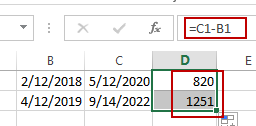# Count the Number of Days between Two Dates

This post will guide you how to count the number of days between two dates in Excel. How to calculate the number of days between 2 dates with a formula in Excel.

## Count the Number of Days between Two Dates

If you want to calculate the number of days between two dates, you need to know the start date and the end date, and you just need to subtract the start date from the date of end.

Assuming that you have a list of data in range B1:C2 that contain two dates, and you want to get the number of days between two dates in B1:C2, and you can use the following formula.

`=C1-B1`

You need to type the formula into a blank cell and press Enter key, and then drag the AutoFill Handle from Cell C1 to C2 to apply this formula.